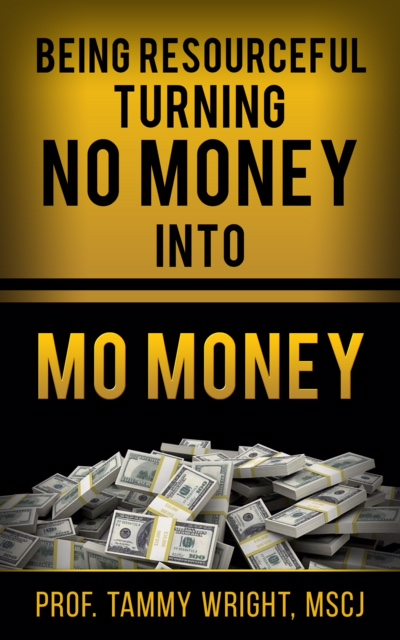Refer a friend and get % off! They'll get % off too.# Being Resourceful Turning no money into mo money

This is an inspiring short informational ebook, gerared twords individuals looking to amplify their income are set to get a thorough lesson in financial ingenuity when you read this book your life will be forever changed. this is a dynamic rsource designed to help individulas tap into their inner talent to become gainfully employed creatively. Using her yeras of experieance of helping others, the authors provides direction and profound insight into diffrent business opportuntiens and ways to make money without spending or having much money.

X
X
X
X
X
X
X
X
X
X
X
X
X
X
X
X
X
X
X
X
X
X
X
X
X
X
X
X
X
X
X
X
X
X
X
X
X
X
X
X
X
X
X
X
X
X
X
X
X
X
X
X
X
X
X
X
X
X
X
X
X
X
X
X
X
X
X
X
X
X
X
X
X
X
X
X
X
X
X
X
X
X
X
X
X
X
X
X
X
X
X
X
X
X
X
X
X

You will get a EPUB (2MB) file

\$ 2.00

\$ 2.00